﻿ Variational Homotopy Perturbation Method for the Nonlinear Generalized Regularized Long Wave Equation

### Variational Homotopy Perturbation Method for the Nonlinear Generalized Regularized Long Wave Equatio...OPEN ACCESSPEER-REVIEWED

## Variational Homotopy Perturbation Method for the Nonlinear Generalized Regularized Long Wave Equation

Amruta Daga1,, Vikas Pradhan1

1Department of Applied Mathematics & Humanities, S.V. National Institute of Technology, Surat, India

### Abstract

This paper presents Variational Homotopy Perturbation method for the nonlinear Generalized Regularized Long Wave (GRLW) equation. The solution of nonlinear GRLW equation is obtained and is solved using the iteration method which is combination of Variational Iteration method and Homotopy Perturbation Method. An example of the propagation of single soliton is given to show the precision of this method.

### At a glance: Figures

12
Prev Next

• Daga, Amruta, and Vikas Pradhan. "Variational Homotopy Perturbation Method for the Nonlinear Generalized Regularized Long Wave Equation." American Journal of Applied Mathematics and Statistics 2.4 (2014): 231-234.
• Daga, A. , & Pradhan, V. (2014). Variational Homotopy Perturbation Method for the Nonlinear Generalized Regularized Long Wave Equation. American Journal of Applied Mathematics and Statistics, 2(4), 231-234.
• Daga, Amruta, and Vikas Pradhan. "Variational Homotopy Perturbation Method for the Nonlinear Generalized Regularized Long Wave Equation." American Journal of Applied Mathematics and Statistics 2, no. 4 (2014): 231-234.

 Import into BibTeX Import into EndNote Import into RefMan Import into RefWorks

### 1. Introduction

The generalized regularized long wave (GRLW) equation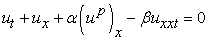(1)

where p is a positive integer, and α and β are positive constants, was proposed many years ago as a model for small-amplitude long waves on the surface of water by Peregrine. The GRLW equation is an alternative to the Korteweg de Vries (KdV) equation for describing nonlinear dispersive waves and can be used to describe phenomena with weak nonlinearity and dispersion waves in fluid mechanics.

For p = 2, Eq. (1) reduces to a special case,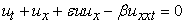(2)

which is called the regularized long wave (RLW) equation, where ε=2α Equation (2) has been studied extensively in the past both analytically and numerically by means of, for example, the Adomian decomposition method, the Fourier method, the finite difference method, finite element method, the variational iteration method, the meshless method and the cubic B-spline collocation procedure etc. Soliman  and E. Yusufoglu  obtained the solution for a short GRLW equation based on the variational iteration method. M. Rafei et al.  obtained solution by HPM. Y.Keskin  studied reduced transform method for solving RLW.  Kaya [4, 5] and A.K. Khalifa [2, 3] implemented the Adomian decomposition method and and the cubic B-spline collocation procedure to obtain the numerical solution of the GRLW equation. Wang Ju-Feng  used Meshless method for nonlinear GRLW. In the present paper Variational Homotopy Perturbation  is elegantly implemented to obtain the solitary wave solution which shows the efficiency of this method with less computational work.

This paper is organized as follows. In Section3, we introduce the Variational Homotopy Perturbation method. In Section 4, the governing equation with initial and boundary conditions are given, VHPM for the problem is obtained. In Section 5, an example which is solved by using the Variational Homotopy Perturbation method in this study and the graphical results of this example are in good agreement with the exact solutions. Section 6 ends this paper with a brief conclusion.

### 2. Variational Iteration and Homotopy Perturbation Method

To illustrate the basic concepts of the VIM and HPM, at first we consider the following nonlinear differential equation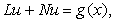(3)

Where

L= A linear operator

N= A nonlinear operator

g(x)= An inhomogeneous term

According to the VIM , we can construct a correction functional as follows: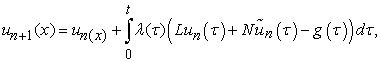(4)

Where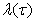is a general lagrange multiplier, which can be identiﬁed optimally via variational theory. The second term on the right hand side is called the correction and is considered as a restricted variation, i.e., δ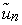= 0. By this method, ﬁrst it is required to determine the lagrange multiplierthat will be identiﬁed optimally. The successive approximations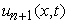, n ≥ 0 of the solution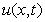will be readily obtained upon using the determined lagrange multiplier and any selective function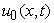. Consequently, the solution is given by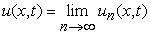(5)

The essential idea of HPM is to introduce a homotopy parameter (say p), which takes the values from 0 to 1. When p = 0, the system of equations is in sufficiently simplified form which normally admits a rather simple solution. As p gradually increases to 1, the system goes through a sequence of “deformation”, the solution of each is “close” to that at the previous stage of “deformation”. Eventually at p = 1, the system takes the original form of equation and the final stage of “deformation” gives the desired solution. To illustrate the basic concept of homotopy perturbation method, we consider the following nonlinear system of differential equations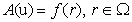(6)

with the boundary conditions: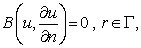(7)

Where

A= A differential operator

B= A boundary operator

f (r)= A known analytic function

Γ= the boundary of the domain Ω

Generally, the operator A can be divided into two parts L and N, where L is a linear operator and N is a nonlinear operator. Therefore equation (7) can be rewritten as follows: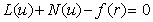(8)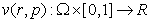Which satisfies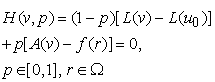(9)

or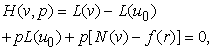(10)

Where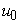is initial approximation of equation (7). In this method, using the homotopy parameter p, we can express in terms of power series as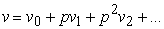(11)

Setting p = 1 yields the approximate solution of equation (11) as below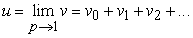(12)

The convergence of series (12) is discussed by Biazar and Ghazvini.

### 3. Variational Homotopy Perturbation Method

In the Homotopy Perturbation Method, the basic assumption is that the solutions can be written in terms of power series in p as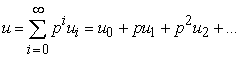(13)

To illustrate the concept of the Variational Homotopy Perturbation Method, we consider the general diﬀerential equation (6). We construct the correction functional equation (7) and apply the Homotopy Perturbation Method to obtain,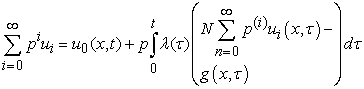(14)

Thus the procedure is formulated by the coupling of variational iteration method and homotopy perturbation method. A comparison of like powers of p gives solutions of various orders.

### 4. The Variational Homotopy Perturbation Method for the GRLW Equation

The GRLW equation in Cartesian coordinates can be written as(15)

where p≥2 is a positive integer, α and β are positive constants.

The boundary and initial conditions are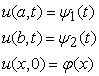Now the Variational Homotopy Perturbation Method can be implemented to obtain the solution of the problem.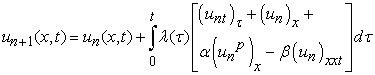(16)

Making the above functional stationary, the Lagrange multiplier can be determined as 𝜆 = −1, which yields the following iteration formula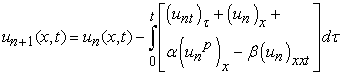(17)

applying Variational Homotopy Perturbation Method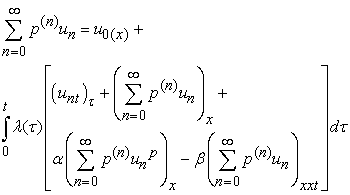(18)

The coefficients of same powers of p are compared by which we get the solutions of various orders.

### 5. Numerical example of Single Soliton

Example We choose the parameter p = 2, i.e. the GRLW equation has the form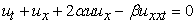(19)

Which is called RLW and has solitary wave solution as follows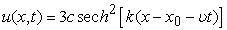(20)

Which represents the motion of a single solitary wave with amplitude 3c, where the wave velocity is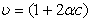(21)

and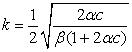(22)

For calculation Purpose let us assume α=0.5, β=1,c=0.1 and x0=0,and k=0.5

The equation (19) becomes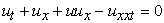(23)

The initial condition becomes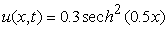(24)

Now the Variational Homotopy Perturbation Method can be implemented we have the correctional function as follows,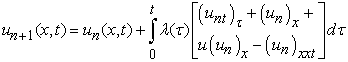(25)

Making the above functional stationary, the Lagrange multiplier can be determined as 𝜆 = −1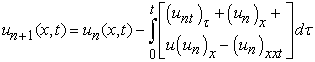(26)

By Variational Homotopy Perturbation Method,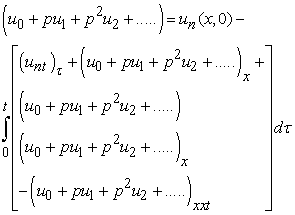(27)
Figure 1. The single solitary wave at t = 0.01, c = 1, δ = 1, μ = 0.125, x0 = 0

Comparing the coefficient of like powers of p, we have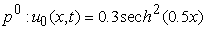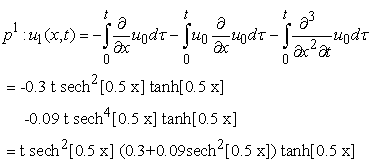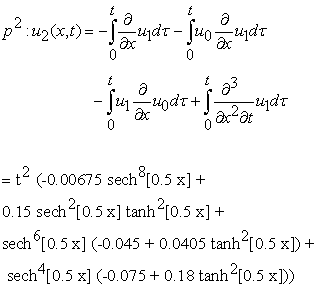And so on.

Thus when we obtain the components the solution becomes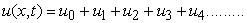(28)

### 6. Conclusions

The main concern of this article is to build an approximate analytical solution for regularized long wave equation. We have achieved this goal by applying reduced differential transform method. The main advantage of the method is the fact that it provides its user with an analytical approximation, in many cases an exact solution, in a rapidly convergent sequence with elegantly computed terms. Graphical representation is presented to show soliton wave Its small size of computation in comparison with the computational size required in other numerical methods, and its rapid convergence show that the method is reliable and introduces a significant improvement in solving partial differential equations over existing methods.

### References

  A.A. Soliman, Numerical simulation of the generalized regularized long wave equation by He’s variational iteration method, Mathematics andComputers in Simulation, 70 (2005) 119-124.In article  A.K. Khalifa, K.R. Raslan, H.M. Alzubaidi, Numerical study using ADM for the modified regularized long wave equation, Applied Mathematical Modelling,32 (2008) 2962-2972.In article CrossRef  A.K. Khalifaa, K.R. Raslan, H.M. Alzubaidi, A collocation method with cubic B-splines for solving the MRLWequation, Journal of Computational and Applied Mathematics, 82 (2005) 369-80.In article  D. Kaya, A numerical simulation of solitary-wave solutions of the generalized regularized long-wave equation, Applied Mathematics and Computation, 149 (2004) 833-841.In article CrossRef  D. Kaya, Salah M.El-Sayed, An application of the decomposition method for the generalized KdV and RLW equations, Chaos, Solitons and Fractals,17 (2003) 869-877.In article CrossRef  D.H. Pregrine, Calculations of the development of an undular bore, J.Fluid Mech, 25 (2003) (1996) 321-330.In article  E. Yusufoglu, A. Bekir, Application of the variational iteration method to the regularized long wave equation, Computers and Mathematics with Applications, 25 (2003) (1996) 321-330.In article  J. H. He, Variational iteration method kind of non-linear analytical technique:Some examples, Int. J. Non-Linear Mech, 34, pp.699-708, 1999.In article CrossRef  M. A. Noor and S. T. Mohyud-Din, Variational homotopy perturbation method for solving higher dimensional initial boundary value problems,Mathematical Problems in Engineering, Article ID 696734, 2008.In article  M. Rafei, D.D. Ganji, H.R. Mohammadi Daniali, H. Pashaei, Application of homotopy perturbation method to the RLW and generalized modified Boussinesq equations, Physics Letters A,364 (2007) 1-6.In article CrossRef  Wang Ju-Feng, Bai Fu-Nong and and Cheng Yu-Min, “A meshless method for the nonlinear generalized regularized long wave equation” Chin. Phys. B Vol. 20, No. 3 (2011).In article  Yildiray Keskin, “Numerical Solution of Regularized Long Wave Equationby Reduced Differential Transform Method”, Applied Mathematical Sciences, Vol. 4, 2010, no. 25, 1221-1231.In article